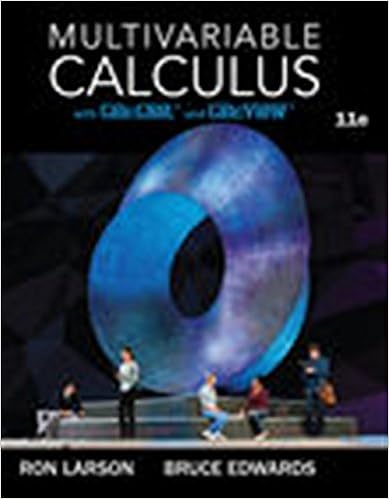# 21C MT2 Study - Co-21C Midterm 2 Review Problems 1 Find the...

• Notes
• 2

This preview shows page 1 - 2 out of 2 pages.

##### We have textbook solutions for you!
The document you are viewing contains questions related to this textbook.The document you are viewing contains questions related to this textbook.
Chapter 11 / Exercise 5
Multivariable Calculus
LarsonExpert Verified
Co-21C Midterm 2 Review Problems 1) Find the interval of convergence for the power series (!n(x!2)nn4nn=12a) Find the 1stfour terms of the Taylor series for f(x)=12centered at a= 3. b) Find the nth degree Taylor series expressed in summation notation. 3) Use the 1st4 terms of the Maclaurin series for exto approximate each value. Then put an upper bound on the error. a) 1eb) e3*Note: Use the fact that e8(why did I choose that fraction?) 4) Use the known Maclaurin series for sinxto approximate xsinx301!to within 1000. 5) Consider the vectors: u!=5i+jand v!=!i+3j. Sketch and find each of the following: a) u!+v!b) v!!u!c) compvud) projvue) orth6) Find a unit vector orthogonal to u= i– 2j+ 37) Find the parametric equation of the line that is perpendicular to the plane x+2y!3z=!4, intersecting it at (4, 5, 6). 8) Consider the three points in space: P(1, 2, 3), Q(6, 5, 4) and R(-2, 0, 1). a) Find the equation of the plane that passes through the points. 1)"#. Be sure to check endpoints! x<27dx1vuk.
##### We have textbook solutions for you!
The document you are viewing contains questions related to this textbook.The document you are viewing contains questions related to this textbook.
Chapter 11 / Exercise 5
Multivariable Calculus
LarsonExpert Verified
•••